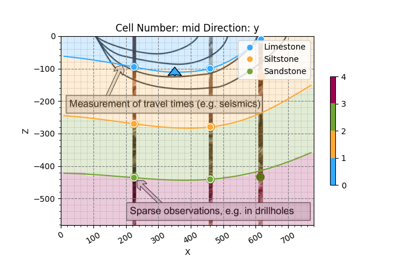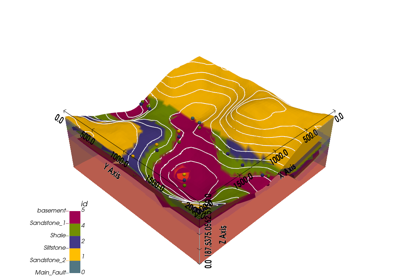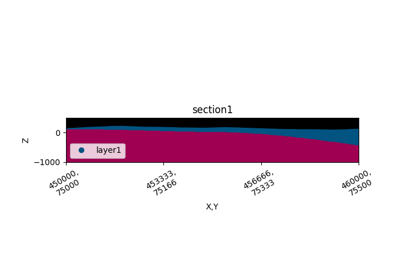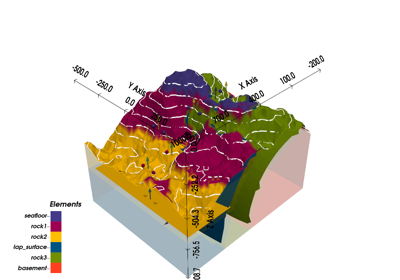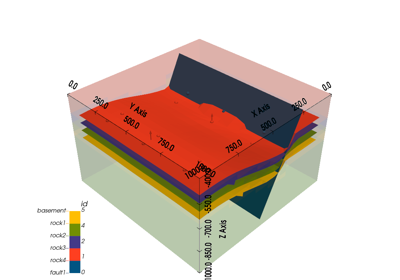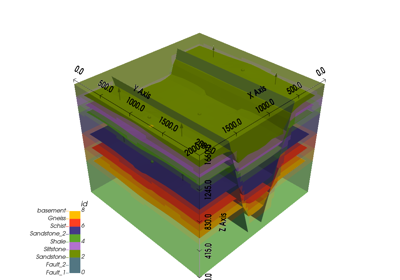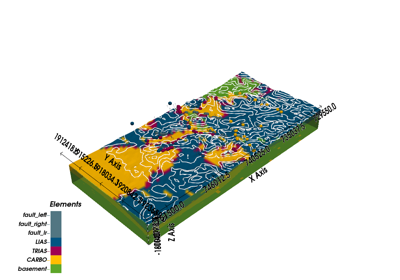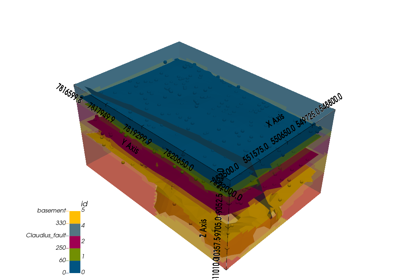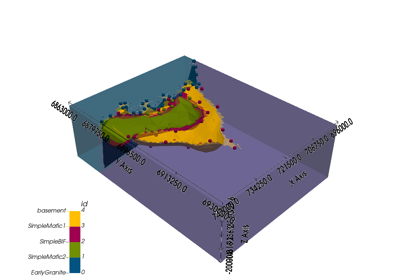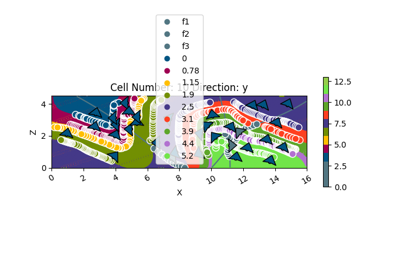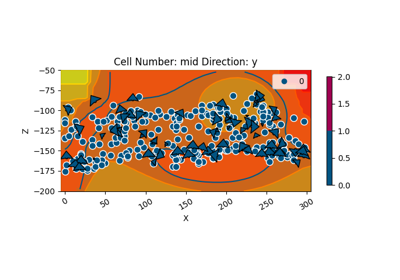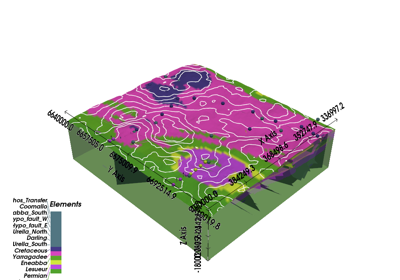# gempy.plot_3d¶

gempy.plot_3d(model, plotter_type='basic', show_data: bool = True, show_results: bool = True, show_surfaces: bool = True, show_lith: bool = True, show_scalar: bool = False, show_boundaries: bool = True, show_topography: Union[bool, list] = False, scalar_field: Optional[str] = None, ve=None, kwargs_plot_structured_grid=None, kwargs_plot_topography=None, kwargs_plot_data=None, image=False, off_screen=False, **kwargs) → gempy.plot.vista.GemPyToVista[source]

foobar

Parameters
• model (gempy.core.model.Project) – Container class of all objects that constitute a GemPy model.

• plotter_type – PyVista plotter types. Supported plotters are: ‘basic’, ‘background’, and ‘notebook’.

• show_data (bool) – Show original input data. Defaults to True.

• show_results (bool) – If False, override show lith, show_scalar, show_values

• show_lith (bool) – Show lithological block volumes. Defaults to True.

• show_scalar (bool) – Show scalar field isolines. Defaults to False.

• show_boundaries (bool) – Show surface boundaries as lines. Defaults to True.

• show_topography (bool) – Show topography on plot. Defaults to False.

• scalar_field (str) – Name of the field to be activated

• series_n (int) – number of the scalar field.

• ve (float) – Vertical Exaggeration

• kwargs_plot_structured_grid

• kwargs_plot_topography

• **kwargs

Returns

gempy.plot.vista.GemPyToVista

## Examples using gempy.plot_3d¶